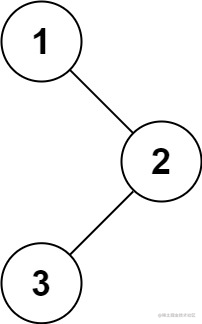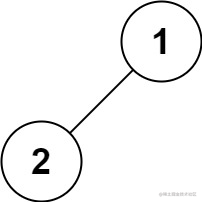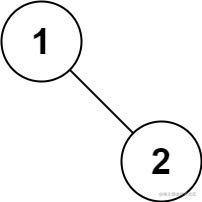# 题目• 输入：`root = [1,null,2,3]`
• 输出：`[1,2,3]`

• 输入：`root = []`
• 输出：`[]`

• 输入：`root = `
• 输出：``• 输入：`root = [1,2]`
• 输出：`[1,2]`• 输入：`root = [1,null,2]`
• 输出：`[1,2]`

# 方法一：递归

## 代码

``````class Solution {
func preorder(_ root: TreeNode?, _ res: inout [Int]) {
guard nil != root else {
return
}
res.append(root!.val)
preorder(root?.left, &res)
preorder(root?.right, &res)
}

func preorderTraversal(_ root: TreeNode?) -> [Int] {
var res: [Int] = []
preorder(root, &res)
return res
}
}

## 复杂度分析

• 时间复杂度：O(n)，其中 n 是二叉树的节点数。每一个节点恰好被遍历一次。

• 空间复杂度：O(n)，为递归过程中栈的开销，平均情况下为 O(log n)，最坏情况下树呈现链状，为 O(n)。

# 方法二：迭代

## 代码

``````class Solution {
func preorderTraversal(_ root: TreeNode?) -> [Int] {
var res: [Int] = []
if nil == root {
return res
}

var stack: [TreeNode?] = []
var node: TreeNode? = root

while !stack.isEmpty || nil != node {
while nil != node {
res.append(node!.val)
stack.append(node)
node = node?.left
}
node = stack.removeLast()?.right
}
return res
}
}

## 复杂度分析

• 时间复杂度：O(n)，其中 n 是二叉树的节点数。每一个节点恰好被遍历一次。

• 空间复杂度：O(n)，为迭代过程中显式栈的开销，平均情况下为 O(log n)，最坏情况下树呈现链状，为 O(n)。

# 方法三：Morris 遍历

## 思路及解法

1. 新建临时节点，令该节点为 `root`
2. 如果当前节点的左子节点为空，将当前节点加入答案，并遍历当前节点的右子节点；
3. 如果当前节点的左子节点不为空，在当前节点的左子树中找到当前节点在中序遍历下的前驱节点：
• 如果前驱节点的右子节点为空，将前驱节点的右子节点设置为当前节点。然后将当前节点加入答案，并将前驱节点的右子节点更新为当前节点。当前节点更新为当前节点的左子节点。
• 如果前驱节点的右子节点为当前节点，将它的右子节点重新设为空。当前节点更新为当前节点的右子节点。
4. 重复步骤 2 和步骤 3，直到遍历结束。

## 代码

``````class Solution {
func preorderTraversal(_ root: TreeNode?) -> [Int] {
var res: [Int] = []
if nil == root {
return res
}

var p1: TreeNode? = root
var p2: TreeNode? = nil
while (nil != p1) {
p2 = p1?.left
if (nil != p2) {
while (nil != p2?.right && p2?.right != p1) {
p2 = p2?.right
}
if (nil == p2?.right) {
res.append(p1!.val)
p2?.right = p1
p1 = p1?.left
continue;
} else {
p2?.right = nil
}
} else {
res.append(p1!.val)
}
p1 = p1?.right
}
return res
}
}

extension TreeNode: Equatable {
public static func == (lhs: TreeNode, rhs: TreeNode) -> Bool {
return lhs === rhs
}
}

## 复杂度分析

• 时间复杂度：O(n)，其中 n 是二叉树的节点数。没有左子树的节点只被访问一次，有左子树的节点被访问两次。

• 空间复杂度：O(1)。只操作已经存在的指针（树的空闲指针），因此只需要常数的额外空间。

iOS